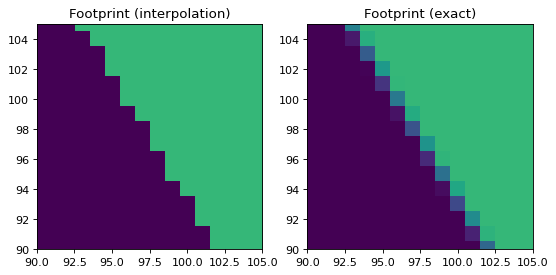# Footprint arrays¶

As described for example in Regular celestial images and cubes, all reprojection functions in this package return a data array (the reprojected values) and a footprint array, which shows which pixels in the new reprojected data fell inside the original image.

For interpolation-based algorithms, the footprint array can either take a value of 0 or 1, but for the ‘exact’ algorithm based on spherical polygon intersection, and in future for the drizzle algorithm, we can actually find out what fraction of the new pixels overlapped with the original image.

To demonstrate this, we take the same example as in the Quick start guide, but this time we reproject the array using both the interpolation and ‘exact’ algorithms, and look closely at what is happening near the boundaries. We start off again by reading in the data:

```from astropy.io import fits
from astropy.utils.data import get_pkg_data_filename
hdu1 = fits.open(get_pkg_data_filename('galactic_center/gc_2mass_k.fits'))
hdu2 = fits.open(get_pkg_data_filename('galactic_center/gc_msx_e.fits'))
```

As before, we now reproject the MSX image to be in the same projection as the 2MASS image, but we do this with two algorithms:

```from reproject import reproject_interp, reproject_exact
```

Finally, we can visualize the footprint, zooming in to one of the edges:

```import matplotlib.pyplot as plt

ax1 = plt.subplot(1,2,1)
ax1.imshow(footprint_interp, origin='lower',
vmin=0, vmax=1.5, interpolation='nearest')
ax1.set_xlim(90, 105)
ax1.set_ylim(90, 105)
ax1.set_title('Footprint (interpolation)')

ax2 = plt.subplot(1,2,2)
ax2.imshow(footprint_exact, origin='lower',
vmin=0, vmax=1.5, interpolation='nearest')
ax2.set_xlim(90, 105)
ax2.set_ylim(90, 105)
ax2.set_title('Footprint (exact)')
```

(png, svg, pdf)As you can see, the footprint for the exact mode correctly shows that some of the new pixels had a fractional overlap with the original image. Note however that this comes at a computational cost, since the exact mode can be significantly slower than interpolation.